## RD Sharma Class 8 Solutions Chapter 3 Squares and Square Roots Ex 3.6

These Solutions are part of RD Sharma Class 8 Solutions. Here we have given RD Sharma Class 8 Solutions Chapter 3 Squares and Square Roots Ex 3.6

Other Exercises

Question 1.
Find the square root of:Solution: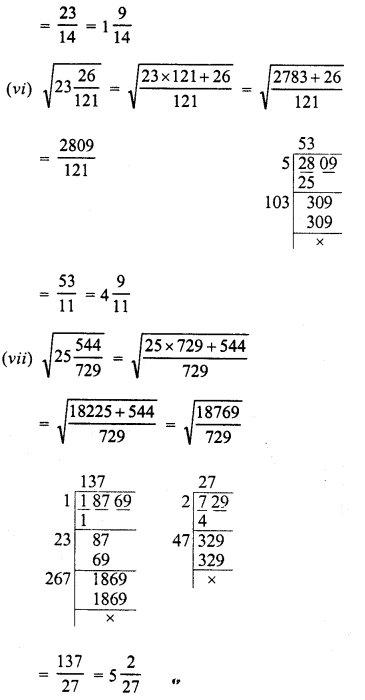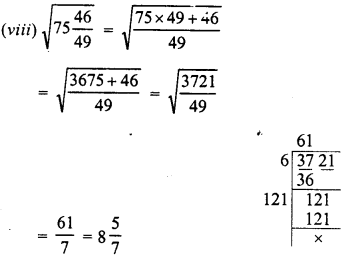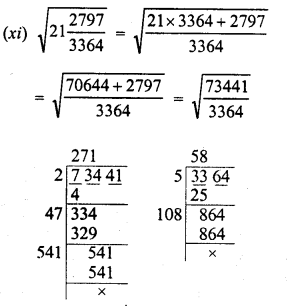Question 2.
Find the value of :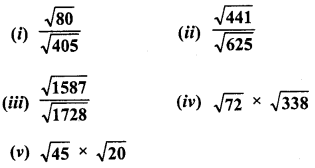Solution: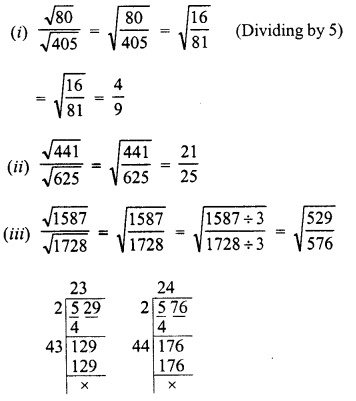Question 3.
The area of a square field is 80 $$\frac {244 }{ 729 }$$ square metres. Find the length of each side of the field.
Solution: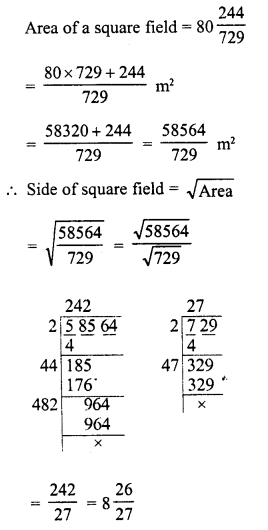Question 4.
The area of a square field is 30 $$\frac {1 }{ 4}$$ m2. Calculate the length of the side of the squre.
Solution:Question 5.
Find the length of a side of a square playground whose area is equal to the area of a rectangular field of dimensions 72 m and 338 m.
Solution:
Length of rectangular field (l) = 338 m
and breadth (b) = 72 m
∴ Area = 1 x 6= 338 x 72 m2
∴ Area of square = 338 x 72 m2 = 24336 m2
and length of the side of the square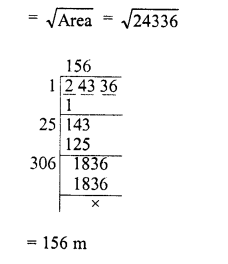Hope given RD Sharma Class 8 Solutions Chapter 3 Squares and Square Roots Ex 3.6 are helpful to complete your math homework.

If you have any doubts, please comment below. Learn Insta try to provide online math tutoring for you.